# 逆向入门01 wp

### 2.逆向工具的基础操作(以解本题为例)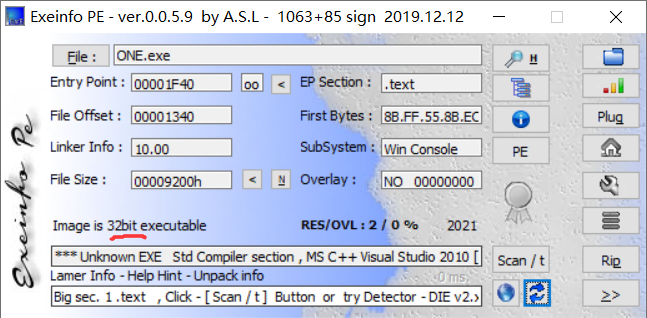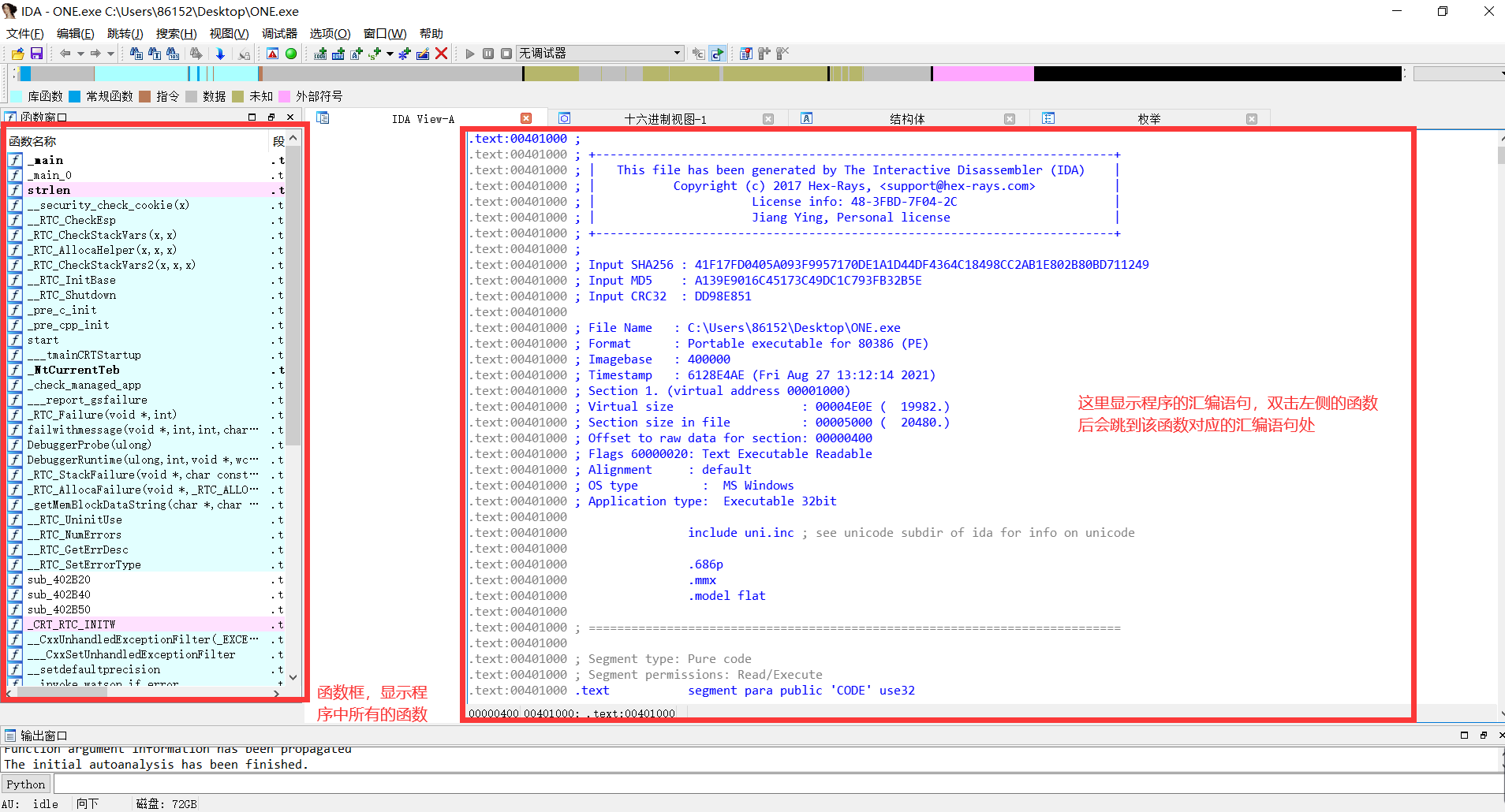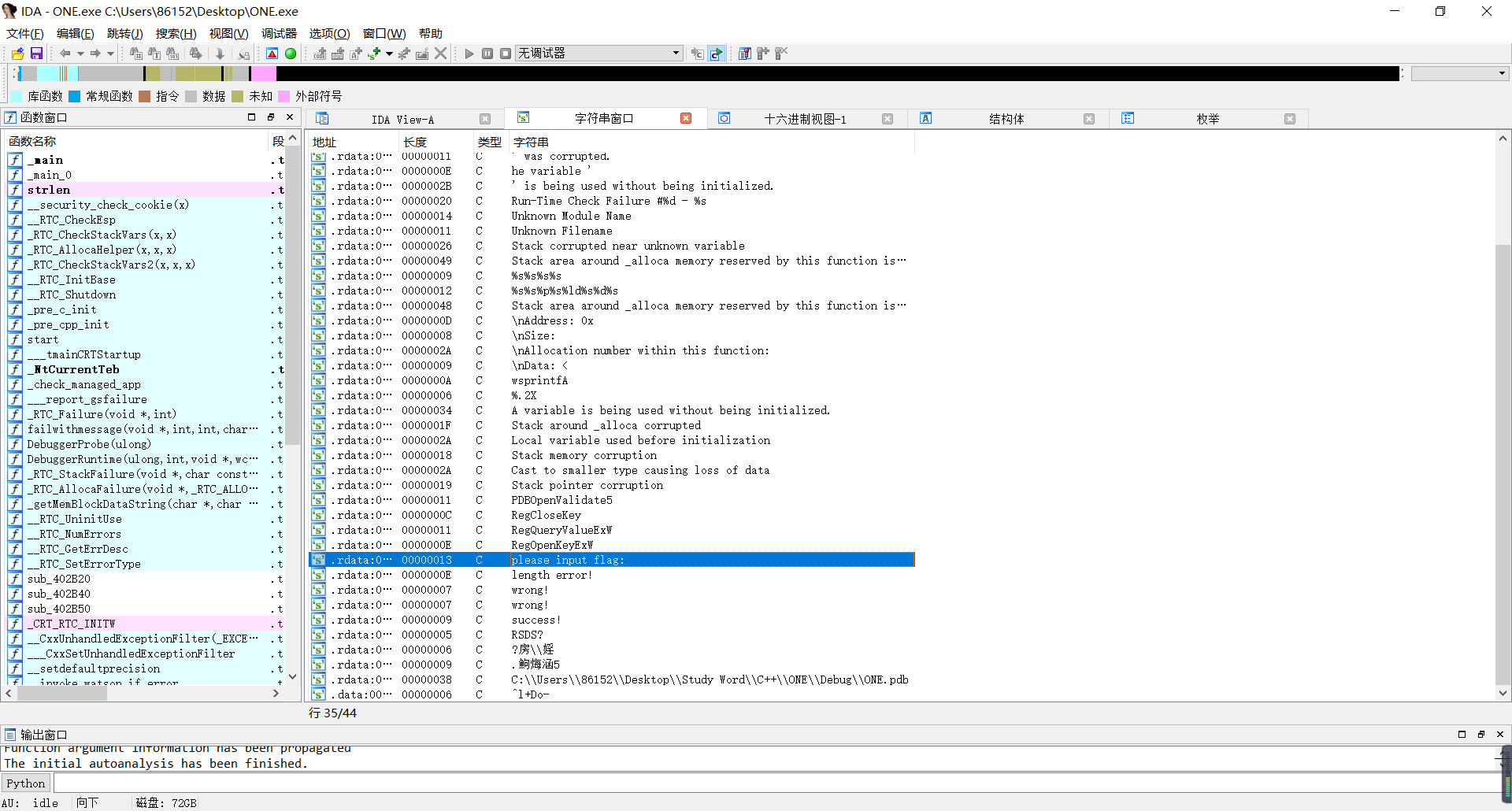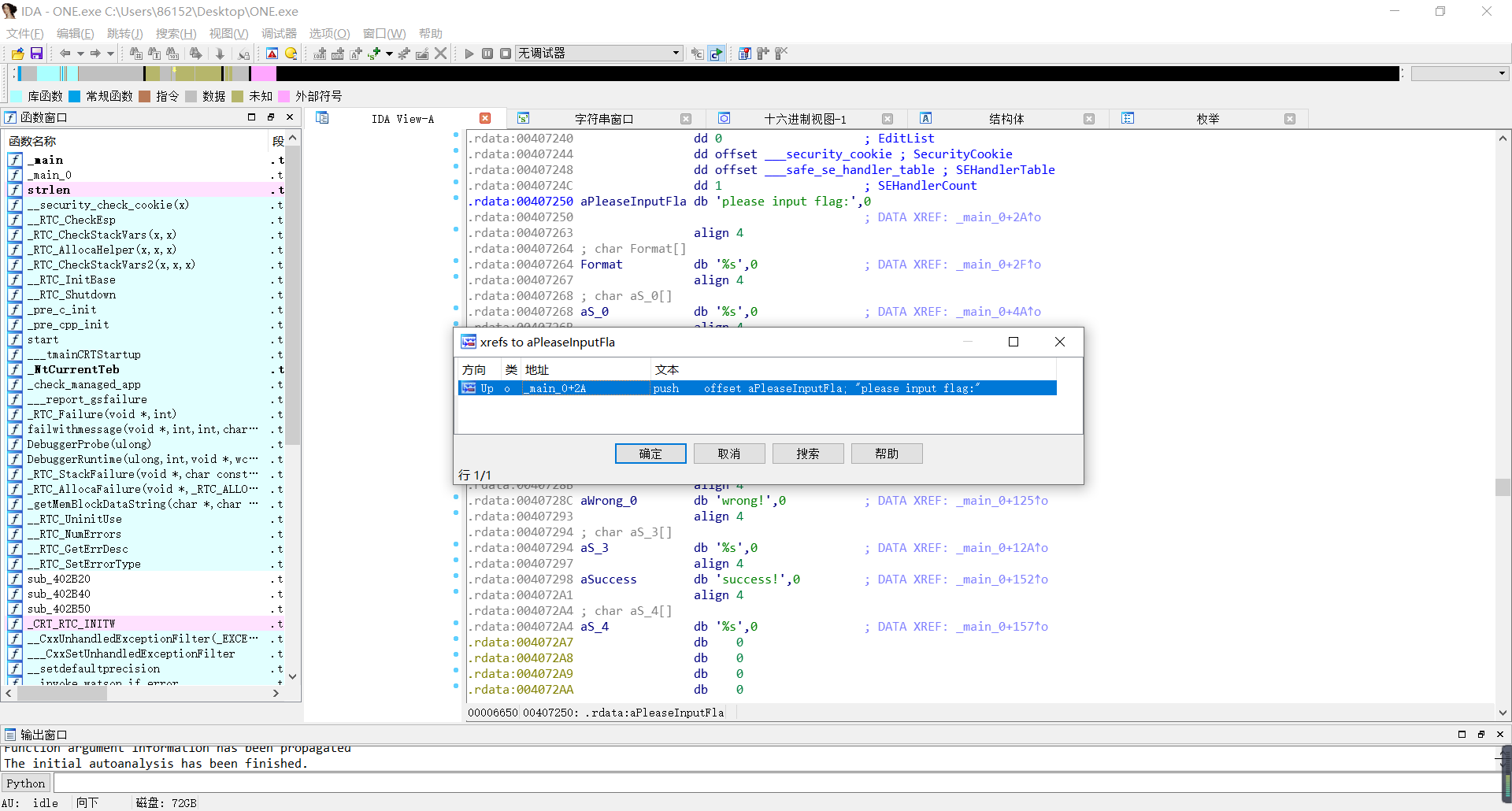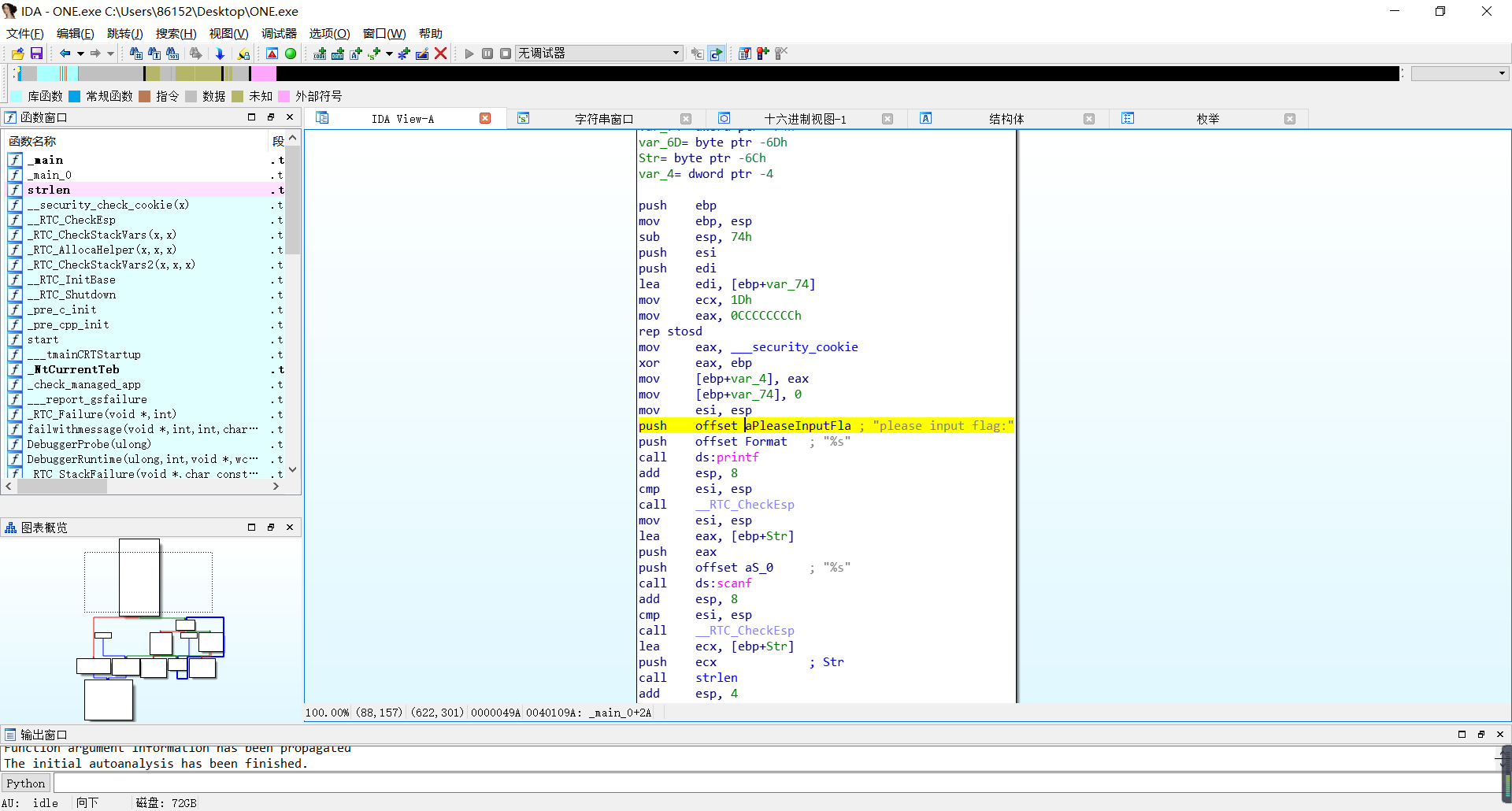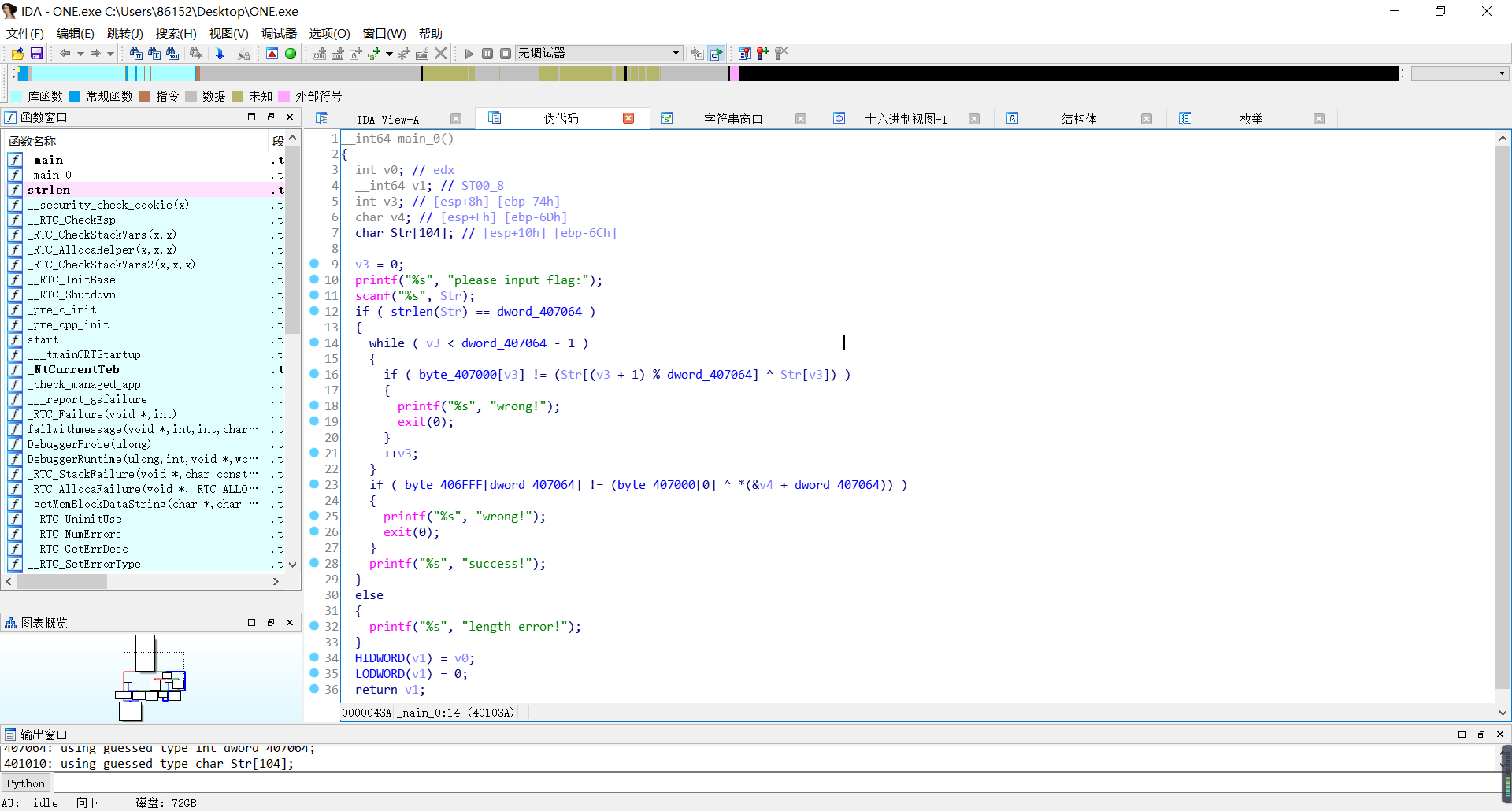``if ( strlen(Str) == dword_407064 )``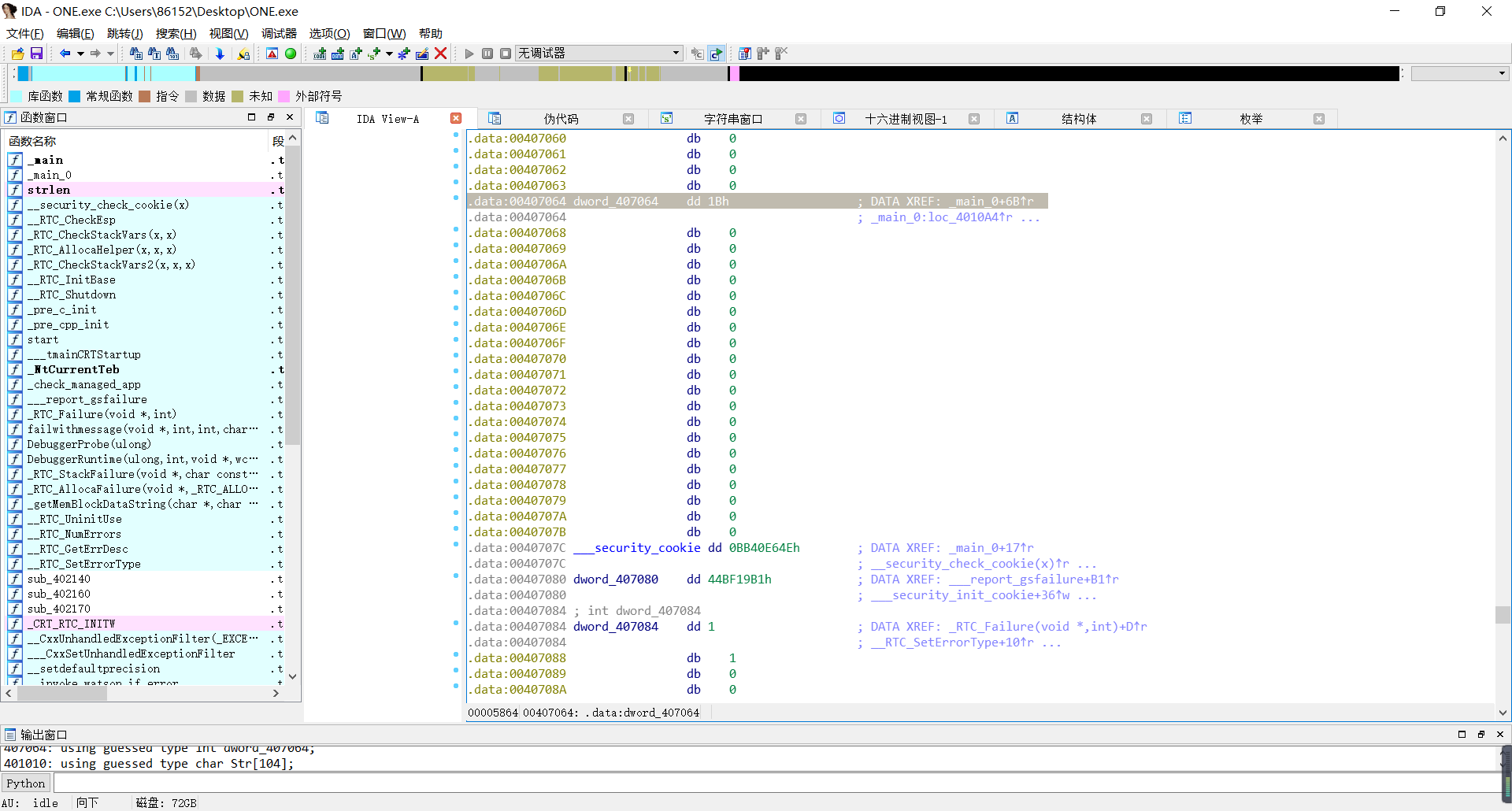``v3 < dword_407064 - 1``

``if ( byte_407000[v3] != (Str[(v3 + 1) % dword_407064] ^ Str[v3]) )``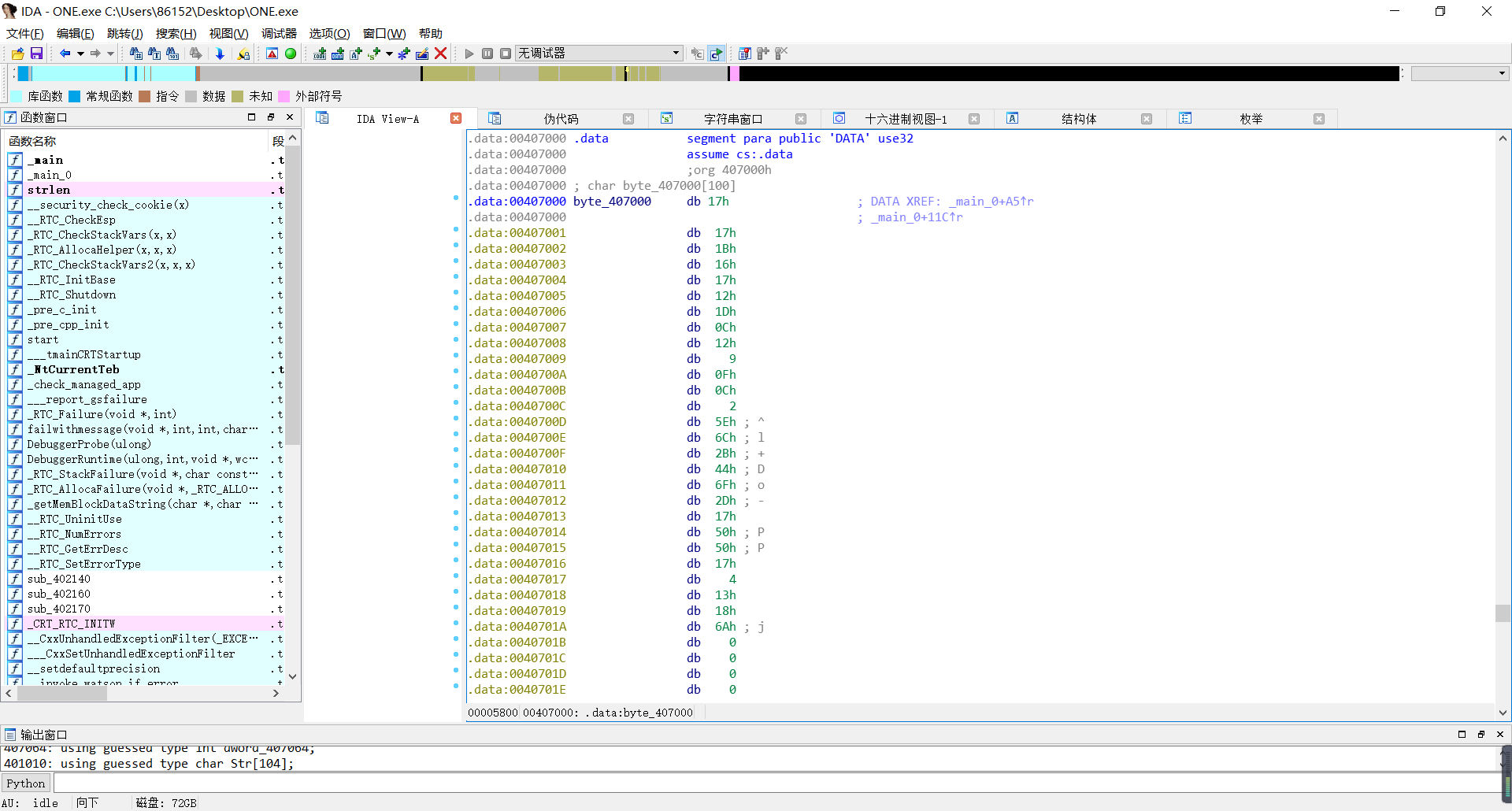``byte_407000[v3] = Str[v3 + 1] ^ Str[v3]) ``

``if ( byte_406FFF[dword_407064] != (byte_407000 ^ *(&v4 + dword_407064)) )``

byte_406FFF与byte_407000的地址相邻，因此byte_406FFF[dword_407064]指向的地址就是byte_407000的最后一位；v4双击后发现地址与Str相邻：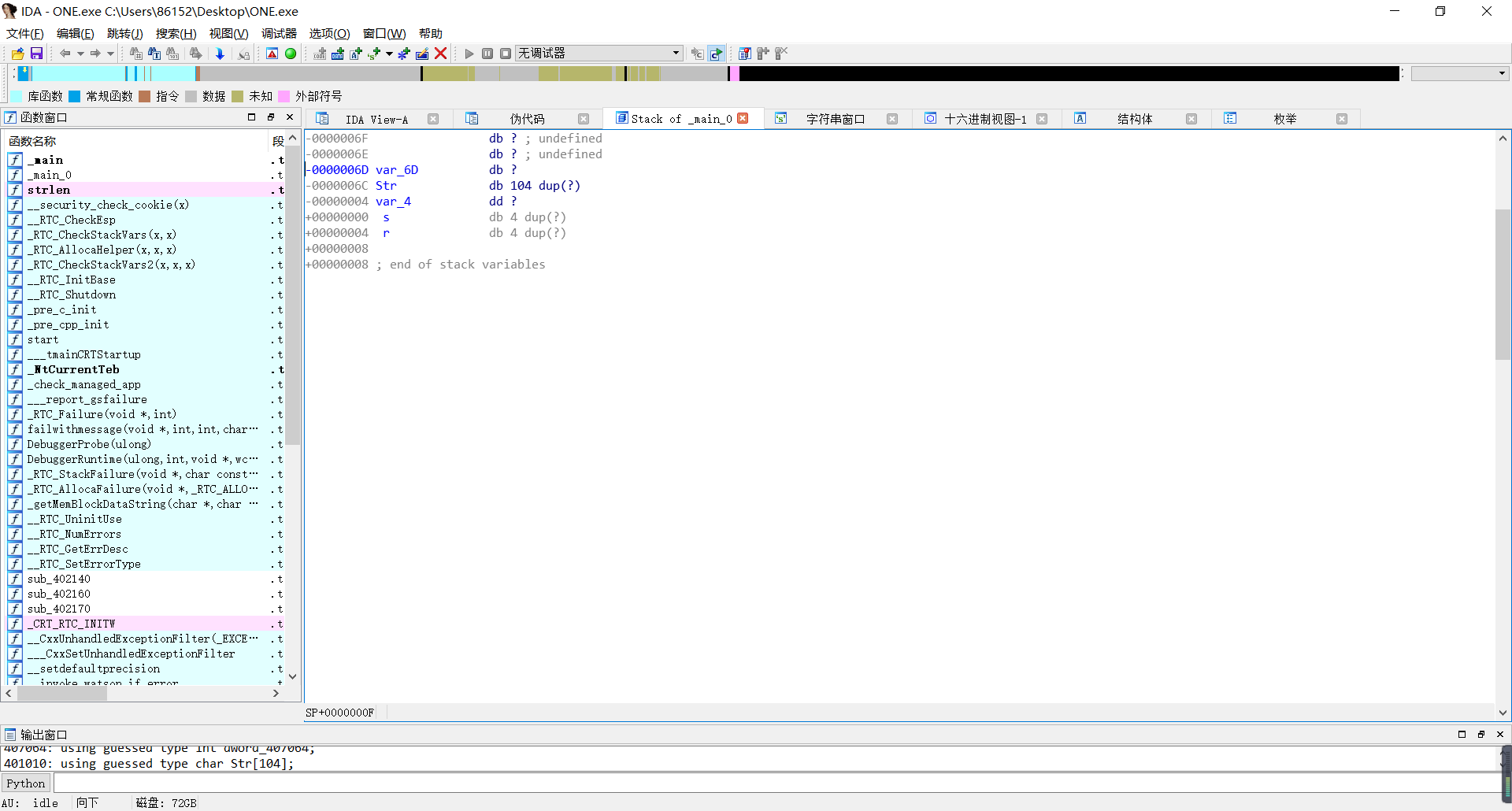Python：

``````list1  = [0x17,0x17,0x1B,0x16,0x17,0x12,0x1D,0x0C,0x12,9,0x0F,0x0C,2,0x5E,0x6C,0x2B,0x44,0x6F,0x2D,0x17,0x50,0x50,0x17,4,0x13,0x18,0x6A]
for i in range(len(list1)-1,-1,-1):
list1[i] = list1[i] ^ list1[ (i+1)%len(list1) ]
list1 = list(map(chr,list1))
print("".join(list1))``````

C++：

``````#include<iostream>
#include<stdlib.h>
#include<cstring>
using namespace std;

int main(){
int array1[] = {0x17,0x17,0x1B,0x16,0x17,0x12,0x1D,0x0C,0x12,9,0x0F,0x0C,2,0x5E,0x6C,0x2B,0x44,0x6F,0x2D,0x17,0x50,0x50,0x17,4,0x13,0x18,0x6A};
for(int i=26;i>-1;i--){ // 解密
array1[i] = array1[i] ^ array1[(i+1) % 27];
}

for(int i=0;i<27;i++){ // 输出
char a = array1[i];
cout<<a;
}

cout<<endl;
system("pause");
return 0;

}``````